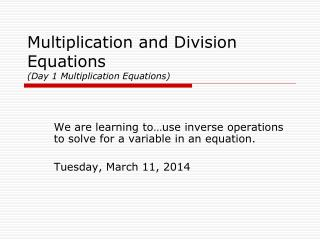DownloadDownload PresentationMultiplication and Division Equations (Day 1 Multiplication Equations)

# Multiplication and Division Equations (Day 1 Multiplication Equations)

Download Presentation## Multiplication and Division Equations (Day 1 Multiplication Equations)

- - - - - - - - - - - - - - - - - - - - - - - - - - - E N D - - - - - - - - - - - - - - - - - - - - - - - - - - -
##### Presentation Transcript

1. Multiplication and Division Equations (Day 1 Multiplication Equations) We are learning to…use inverse operations to solve for a variable in an equation. Tuesday, March 11, 2014

2. Find the following Algebra Tiles… Trace each of these Algebra Tiles on your notes. Name each tile by its area. The red side of each tile represents… one negative unit! 1 unit x units y units 1 unit Area/Name: “1 UNIT TILE” The other (“non-red”) side of the tile represents… one positive unit! 1 unit Area/Name: “X TILE” 1 unit Area/Name: “Y UNIT TILE”

3. Multiplication and Division Equations • The variables, x and y, represent missing values in our equations today. • Our job is to find the value of the variable by isolating the variable on one side of the equation.

7. Example #4 Record your steps mathematically: y y = Check your Solution:

8. Example #5 Record your steps mathematically: x x = x Check your Solution:

9. Team Practice • With your team: • Set up each equation on your Equation Mat using your set of Algebra Tiles. • Draw what you see on your Equation Mat as you solve each equation. • Record your equation solving steps mathematically. • Check your solutions by using substitution.

10. One-Step Multiplication and Division Equations (Day 2 Division Equations) We are learning to…use inverse operations to solve for a variable in an equation. Tuesday, March 11, 2014

11. Multiplication and Division Equations • The variables, x and y, represent missing values in our equations today. • Our job is to find the value of the variable by isolating the variable on one side of the equation.

12. Example #1 Record your steps mathematically: 1/2x = x 1/2x Check your Solution:

13. Example #2 Record your steps mathematically: 1/3y 1/3y = y Check your Solution: 1/3y

14. Example #3 Record your steps mathematically: 1/5x 1/5x = x -x 1/5x Check your Solution: 1/5x 1/5x

15. Example #4 Record your steps mathematically: 1/4y 1/4y = -y y 1/4y Check your Solution: 1/4y

16. Team Practice • With your team: • Set up each equation on your Equation Mat using your set of Algebra Tiles. • Draw what you see on your Equation Mat as you solve each equation. • Record your equation solving steps mathematically. • Check your solutions by using substitution.